# RD Sharma Solutions for Class 9 Maths Chapter 10 Congruent Triangles - Free PDF Download# RD Sharma Solutions for Class 9 Maths Chapter 10 Congruent Triangles

Hey, are you a class 9 student and looking for ways to download RD Sharma Solutions for Class 9 Maths Chapter 10 "Congruent Triangles"? If yes. Then read this post till the end.

In this article, we have listed RD Sharma Solutions for Class 9 Maths Chapter 10 in PDF that is prepared by Kota’s top IITian’s Faculties by keeping Simplicity in mind.

If you want to learn and understand class 9 Maths Chapter 10 "Congruent Triangles" in an easy way then you can use these solutions PDF.

Chapter 10 of RD Sharma Class 9 deals with Congruent Triangles, their different types and formulas. If you want to improve your basic in Congruent Triangles, then you can use this.

RD Sharma Solutions helps students to Practice important concepts of subjects easily. RD Sharma class 9 solutions provide detailed explanations of all the  exercise questions that students can use to clear their doubts instantly.

If you want to become good at Math then it is very important for you to have a good knowledge of all the important topics of class 9 math, so to learn and practice those topics you can use eSaral RD Sharma Solutions.

So, without wasting more time Let’s start.

## RD Sharma Solutions for Class 9 Maths Chapter 10 Congruent Triangles - Free PDF Download

Question 1: In figure, the sides BA and CA have been produced such that $B A=A D$ and $C A=$ AE. Prove that segment DE ॥ BC.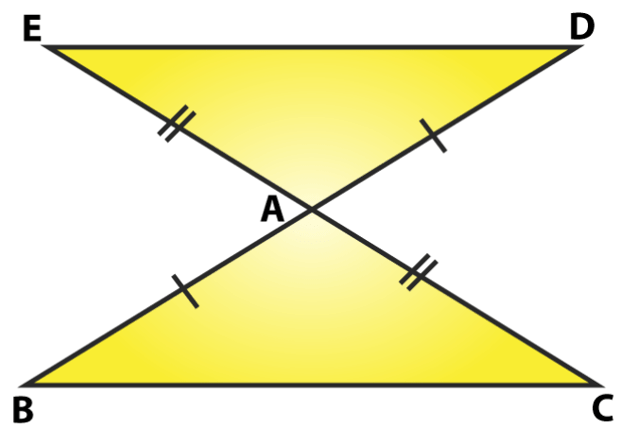Solution: Sides $B A$ and $C A$ have been produced such that $B A=A D$ and $C A=A E$.

To prove: DE ॥ BC

Consider $\triangle \mathrm{BAC}$ and $\triangle \mathrm{DAE}$

$\mathrm{BA}=\mathrm{AD}$ and $\mathrm{CA}=\mathrm{AE}$ (Given)

$\angle B A C=\angle D A E$ (vertically opposite angles)

By SAS congruence criterion, we have

$\triangle \mathrm{BAC} \simeq \triangle \mathrm{DAE}$

We know, corresponding parts of congruent triangles are equal

So, $\mathrm{BC}=\mathrm{DE}$ and $\angle \mathrm{DEA}=\angle \mathrm{BCA}, \angle \mathrm{EDA}=\angle \mathrm{CBA}$

Now, DE and BC are two lines intersected by a transversal DB s.t.

$\angle \mathrm{DEA}=\angle \mathrm{BCA}$ (alternate angles are equal)

Therefore, DE ॥ BC. Proved.

Question 2: In a PQR, if $P Q=Q R$ and $L, M$ and $N$ are the mid-points of the sides $P Q, Q R$ and RP respectively. Prove that $L N=M N$.

Solution: Draw a figure based on given instruction,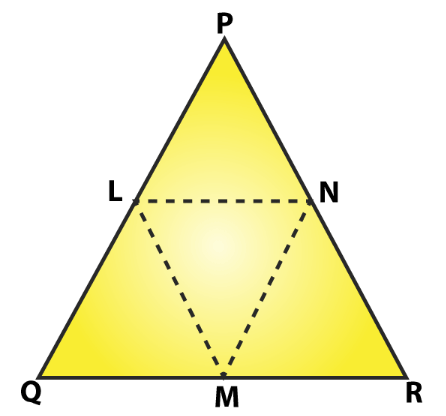In $\triangle \mathrm{PQR}, \mathrm{PQ}=\mathrm{QR}$ and $\mathrm{L}, \mathrm{M}, \mathrm{N}$ are midpoints of the sides $\mathrm{PQ}, \mathrm{QP}$ and $\mathrm{RP}$ respectively (Given)

To prove : $L N=M N$

As two sides of the triangle are equal, so $\triangle \mathrm{PQR}$ is an isosceles triangle

$\mathrm{PQ}=\mathrm{QR}$ and $\angle \mathrm{QPR}=\angle \mathrm{QRP} \ldots \ldots$ (i)

Also, $L$ and $M$ are midpoints of $P Q$ and $Q R$ respectively

$\mathrm{PL}=\mathrm{LQ}=\mathrm{QM}=\mathrm{MR}=\mathrm{QR} / 2$

Now, consider $\triangle$ LPN and $\Delta$ MRN,

$\mathrm{LP}=\mathrm{MR}$

$\angle \mathrm{LPN}=\angle \mathrm{MRN}$ [From (i)]

$\angle \mathrm{QPR}=\angle \mathrm{LPN}$ and $\angle \mathrm{QRP}=\angle \mathrm{MRN}$

$\mathrm{PN}=\mathrm{NR}[\mathrm{N}$ is midpoint of $\mathrm{PR}]$

By SAS congruence criterion,

$\Delta \mathrm{LPN} \simeq \triangle \mathrm{MRN}$

We know, corresponding parts of congruent triangles are equal.

So $L N=M N$

Proved.

Question 3: In figure, PQRS is a square and SRT is an equilateral triangle. Prove that

(i) $P T=Q T$ (ii) $\angle T Q R=15^{\circ}$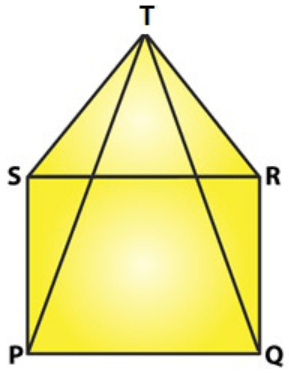Solution: Given: PQRS is a square and SRT is an equilateral triangle.

To prove:

(i) $\mathrm{PT}=\mathrm{QT}$ and (ii) $\angle \mathrm{TQR}=15^{\circ}$

Now,

PQRS is a square:

$\mathrm{PQ}=\mathrm{QR}=\mathrm{RS}=\mathrm{SP} \ldots$(i)

And $\angle S P Q=\angle P Q R=\angle Q R S=\angle R S P=90^{\circ}$

Also, $\triangle$ SRT is an equilateral triangle:

$\mathrm{SR}=\mathrm{R} \mathrm{T}=\mathrm{TS}$.$\ldots \ldots$ (ii)

And $\angle T S R=\angle S R T=\angle R T S=60^{\circ}$

From (i) and (ii)

$\mathrm{PQ}=\mathrm{QR}=\mathrm{SP}=\mathrm{SR}=\mathrm{RT}=\mathrm{TS} \ldots \ldots$(iii)

From figure,

$\angle T S P=\angle T S R+\angle R S P$

$=60^{\circ}+90^{\circ}=150^{\circ}$ and

$\angle \mathrm{TRQ}=\angle \mathrm{TRS}+\angle \mathrm{SRQ}$

$=60^{\circ}+90^{\circ}=150^{\circ}$

$\Rightarrow \angle \mathrm{TSP}=\angle \mathrm{TRQ}=150^{\circ} \ldots \ldots \ldots \ldots \ldots \ldots .$ (iv)

By SAS congruence criterion, $\triangle$ TSP $\simeq \triangle$ TRQ

We know, corresponding parts of congruent triangles are equal

So, $\mathrm{PT}=\mathrm{QT}$

Proved part (i).

Now, consider $\Delta$ TQR.

$\mathrm{QR}=$ TR [From (iii)]

$\triangle \mathrm{TQR}$ is an isosceles triangle.

$\angle \mathrm{QTR}=\angle \mathrm{TQR}$ [angles opposite to equal sides $]$

Sum of angles in a triangle $=180$ 。

$\Rightarrow \angle Q T R+\angle T Q R+\angle T R Q=180^{\circ}$

$\Rightarrow 2 \angle \mathrm{TQR}+150^{\circ}=180^{\circ}[\mathrm{From}(\mathrm{iv})]$

$=>2 \angle \mathrm{TQR}=30^{\circ}$

$=>\angle \mathrm{TQR}=15^{\circ}$

Hence proved part (ii).

Question 4: Prove that the medians of an equilateral triangle are equal.

Solution: Consider an equilateral $\triangle \mathrm{ABC}$, and Let $\mathrm{D}, \mathrm{E}, \mathrm{F}$ are midpoints of $\mathrm{BC}, \mathrm{CA}$ and $\mathrm{AB}$.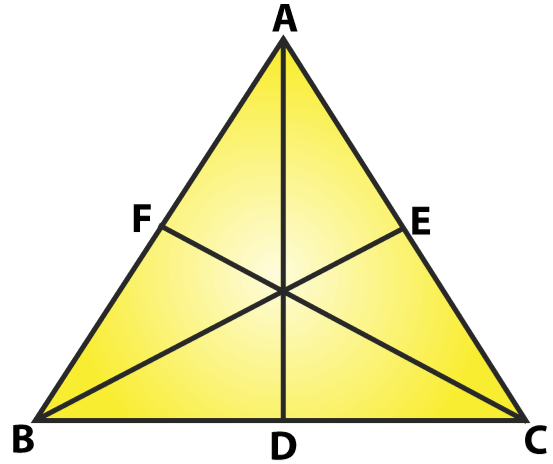Here, $A D, B E$ and $C F$ are medians of $\triangle A B C$.

Now,

$D$ is midpoint of $B C=>B D=D C$

Similarly. $C E=E A$ and $A F=F B$

Since $\triangle \mathrm{ABC}$ is an equilateral triangle

$\mathrm{AB}=\mathrm{BC}=\mathrm{CA} \ldots$. (i)

$B D=D C=C E=E A=A F=F B \ldots \ldots \ldots$.(ii)

And also, $\angle \mathrm{ABC}=\angle \mathrm{BCA}=\angle \mathrm{CAB}=60^{\circ} \ldots \ldots \ldots$(iii)

Consider $\triangle \mathrm{ABD}$ and $\triangle \mathrm{BCE}$

$A B=B C$ [From (i)]

$\mathrm{BD}=\mathrm{CE}$ [From (ii) $]$

$\angle \mathrm{ABD}=\angle \mathrm{BCE}$ [From (iii)]

By SAS congruence criterion,

$\triangle \mathrm{ABD} \simeq \triangle \mathrm{BCE}$

$\Rightarrow A D=B E \ldots \ldots$(iv)

[Corresponding parts of congruent triangles are equal in measure]

Now, consider $\triangle \mathrm{BCE}$ and $\triangle \mathrm{CAF}$,

$\mathrm{BC}=\mathrm{CA}$ [From (i)]

$\angle B C E=\angle$ CAF [From (iii)]

$\mathrm{CE}=\mathrm{AF}[\mathrm{From}$ (ii) $]$’

By SAS congruence criterion,

$\triangle \mathrm{BCE} \simeq \triangle \mathrm{CAF}$

$=>\mathrm{BE}=\mathrm{CF} \ldots \ldots$(v)

[Corresponding parts of congruent triangles are equal] From (iv) and (v), we have

$A D=B E=C F$

Median $\mathrm{AD}=$ Median $\mathrm{BE}=$ Median $\mathrm{CF}$

The medians of an equilateral triangle are equal.

Hence proved

Question 5: In a $\triangle \mathrm{ABC}$, if $\angle \mathrm{A}=120^{\circ}$ and $\mathrm{AB}=\mathrm{AC}$. Find $\angle \mathrm{B}$ and $\angle \mathrm{C}$.

Solution: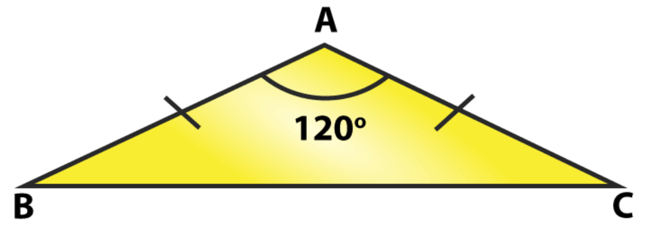To find: $\angle B$ and $\angle C$.

Here, $\triangle \mathrm{ABC}$ is an isosceles triangle since $\mathrm{AB}=\mathrm{AC}$

$\angle B=\angle C \ldots \ldots \ldots$…… (i)

[Angles opposite to equal sides are equal]

We know, sum of angles in a triangle $=180^{\circ}$

$\angle A+\angle B+\angle C=180^{\circ}$

$\angle A+\angle B+\angle B=180^{\circ}$ (using (i)

$120^{\circ}+2 \angle B=180^{\circ}$

$2 \angle B=180^{\circ}-120^{\circ}=60^{\circ}$

$\angle B=30^{\circ}$

Therefore, $\angle B=\angle C=30^{\circ}$

Question 6: In a $\triangle A B C$, if $A B=A C$ and $\angle B=70^{\circ}$, find $\angle A$.

Solution: Given: In a $\triangle A B C, A B=A C$ and $\angle B=70^{\circ}$

$\angle B=\angle C$ [Angles opposite to equal sides are equal]

Therefore, $\angle B=\angle C=70^{\circ}$

Sum of angles in a triangle $=180^{\circ}$

$\angle A+\angle B+\angle C=180^{\circ}$

$\angle A+70^{\circ}+70^{\circ}=180^{\circ}$

$\angle A=180^{\circ}-140^{\circ}$

$\angle A=40^{\circ}$

Exercise $10.2$

Question 1: In figure, it is given that $\mathrm{RT}=\mathrm{TS}, \angle 1=2 \angle 2$ and $\angle 4=2(\angle 3)$. Prove that $\Delta \mathrm{RBT} \cong$ \DeltaSAT.Solution: In the figure,

$\mathrm{RT}=\mathrm{TS} \ldots \ldots(\mathrm{i})$

$\angle 1=2 \angle 2 \ldots \ldots$(ii)

And $\angle 4=2<3 \ldots \ldots$(iii)

To prove: $\Delta \mathrm{RBT} \cong \Delta \mathrm{SAT}$

Let the point of intersection $\mathrm{RB}$ and SA be denoted by O

$\angle \mathrm{AOR}=\angle \mathrm{BOS}$ [Vertically opposite angles $]$

or $\angle 1=\angle 4$

$2 \angle 2=2 \angle 3$ [From (ii) and (iii)]

or $\angle 2=\angle 3 \ldots \ldots$(iv)

Now in $\Delta$ TRS, we have $\mathrm{RT}=\mathrm{TS}$

$\Rightarrow \Delta \mathrm{TRS}$ is an isosceles triangle

$\angle \mathrm{TRS}=\angle \mathrm{TSR} \ldots \ldots(\mathrm{v})$

But, $\angle$ TRS $=\angle T R B+\angle 2 \ldots \ldots$ (vi)

$\angle \mathrm{TSR}=\angle \mathrm{TSA}+\angle 3 \ldots \ldots(\mathrm{vii})$

Putting (vi) and (vii) in (v) we get

$\angle T R B+\angle 2=\angle T S A+\angle 3$

$\Rightarrow \angle$ TRB $=\angle$ TSA [From (iv)]

Consider $\triangle$ RBT and $\triangle$ SAT

$\mathrm{RT}=\mathrm{ST}[$ From (i) $]$

$\angle \mathrm{TRB}=\angle \mathrm{TSA}[\mathrm{From}(\mathrm{iv})]$

By ASA criterion of congruence, we have

$\Delta \mathrm{RBT} \cong \Delta \mathrm{SAT}$

Question 2: Two lines AB and CD intersect at O such that BC is equal and parallel to AD. Prove that the lines $\mathrm{AB}$ and $\mathrm{CD}$ bisect at $\mathrm{O} .$

Solution: Lines $A B$ and $C D$ Intersect at $O$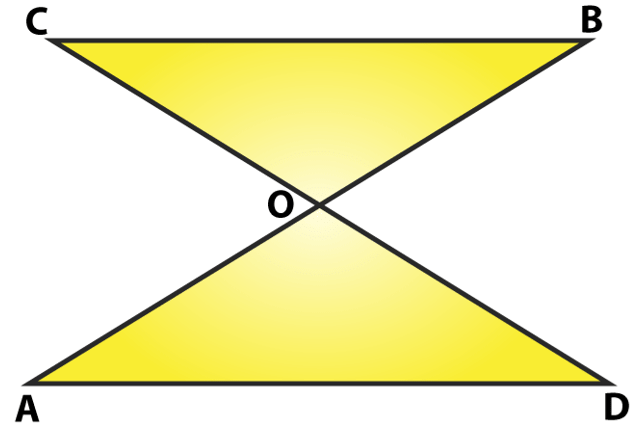Such that BC $\|$ AD and

$\mathrm{BC}=\mathrm{AD} \ldots \ldots \text {.(i) }$

To prove : $A B$ and $C D$ bisect at $O$.

First we have to prove that $\triangle \mathrm{AOD} \cong \Delta \mathrm{BOC}$

$\angle \mathrm{OCB}=\angle \mathrm{ODA}[\mathrm{AD} \| \mathrm{BC}$ and $\mathrm{CD}$ is transversal]

$\mathrm{AD}=\mathrm{BC}[$ from $(\mathrm{i})]$

$\angle \mathrm{OBC}=\angle \mathrm{OAD}[\mathrm{AD} \| \mathrm{BC}$ and $\mathrm{AB}$ is transversal]

By ASA Criterion:

$\triangle \mathrm{AOD} \cong \Delta \mathrm{BOC}$

$\mathrm{OA}=\mathrm{OB}$ and $\mathrm{OD}=\mathrm{OC}$ (By c.p.c.t.)

Therefore, $A B$ and $C D$ bisect each other at $O$.

Hence Proved.

Question 3: BD and CE are bisectors of $\angle B$ and $\angle C$ of an isosceles $\triangle A B C$ with $A B=A C$. Prove that $B D=C E$.

Solution: $\triangle A B C$ is isosceles with $A B=A C$ and $B D$ and $C E$ are bisectors of $\angle B$ and $\angle C$ We have to prove $B D$ $=\mathrm{CE}$. (Given)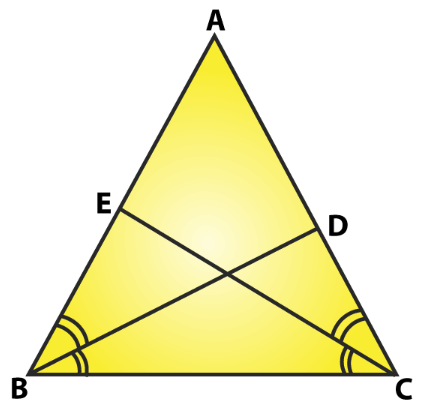Since $\mathrm{AB}=\mathrm{AC}$

$\Rightarrow \angle \mathrm{ABC}=\angle \mathrm{ACB} \ldots \ldots(\mathrm{i})$

[Angles opposite to equal sides are equal]

Since $\mathrm{BD}$ and $\mathrm{CE}$ are bisectors of $\angle \mathrm{B}$ and $\angle \mathrm{C}$

$\angle A B D=\angle D B C=\angle B C E=E C A=\angle B / 2=\angle C / 2$...(ii)

Now, Consider $\Delta \mathrm{EBC}=\Delta \mathrm{DCB}$

$\angle E B C=\angle D C B$ [From (i)]

$\mathrm{BC}=\mathrm{BC}$ [Common side]

$\angle \mathrm{BCE}=\angle \mathrm{CBD}$ [From (ii)]

By ASA congruence criterion, $\triangle \mathrm{EBC} \cong \triangle \mathrm{DCB}$

Since corresponding parts of congruent triangles are equal.

$=>\mathrm{CE}=\mathrm{BD}$

or, $B D=C E$

Hence proved.

Exercise $10.3$

Question 1: In two right triangles one side an acute angle of one are equal to the corresponding side and angle of the other. Prove that the triangles are congruent.

Solution: In two right triangles one side and acute angle of one are equal to the corresponding side and angles of the other. (Given)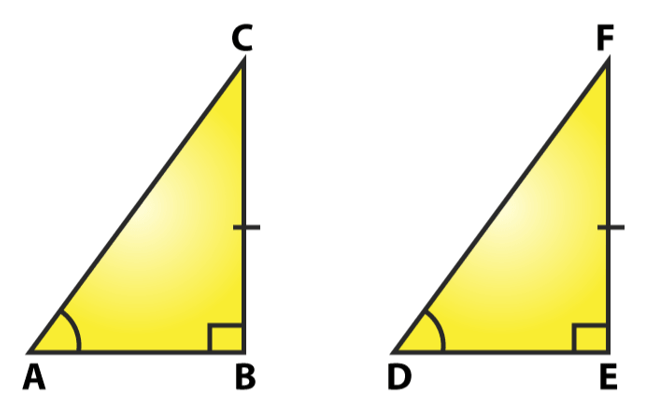To prove: Both the triangles are congruent.

Consider two right triangles such that

$\angle B=\angle E=90^{\circ} \ldots \ldots$(i)

$\mathrm{AB}=\mathrm{DE} \ldots$(ii)

$\angle C=\angle F \ldots \ldots$ ……(iii)

Here we have two right triangles, $\triangle \mathrm{ABC}$ and $\triangle \mathrm{DEF}$

From (i), (ii) and (iii),

By AAS congruence criterion, we have $\triangle \mathrm{ABC} \cong \Delta \mathrm{DEF}$

Both the triangles are congruent. Hence proved.

Question 2: If the bisector of the exterior vertical angle of a triangle be parallel to the base. Show that the triangle is isosceles.

Solution: Let $\mathrm{ABC}$ be a triangle such that $\mathrm{AD}$ is the angular bisector of exterior vertical angle, $\angle \mathrm{EAC}$ and $\mathrm{AD}$ ॥ BC.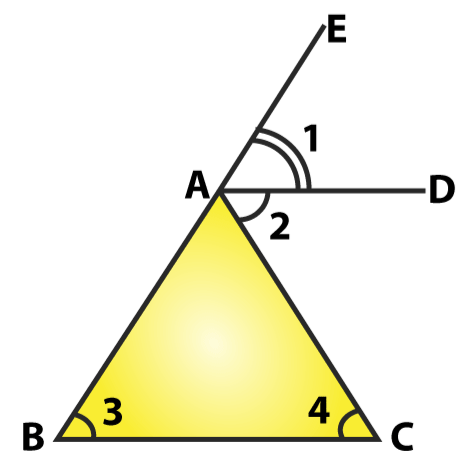From figure,

$\angle 1=\angle 2[\mathrm{AD}$ is a bisector of $\angle \mathrm{EAC}]$

$\angle 1=\angle 3$ [Corresponding angles]

and $\angle 2=\angle 4$ [alternative angle]

From above, we have $\angle 3=\angle 4$

This implies, $A B=A C$

Two sides $A B$ and $A C$ are equal.

$=>\triangle \mathrm{ABC}$ is an isosceles triangle.

Question 3: In an isosceles triangle, if the vertex angle is twice the sum of the base angles, calculate the angles of the triangle.

Solution: Let $\triangle \mathrm{ABC}$ be isosceles where $\mathrm{AB}=\mathrm{AC}$ and $\angle \mathrm{B}=\angle \mathrm{C}$

Given: Vertex angle $\mathrm{A}$ is twice the sum of the base angles $\mathrm{B}$ and $\mathrm{C}$. i.e., $\angle \mathrm{A}=2(\angle \mathrm{B}+\angle \mathrm{C})$

$\angle A=2(\angle B+\angle B)$

$\angle A=2(2 \angle B)$

$\angle A=4(\angle B)$

Now, We know that sum of angles in a triangle $=180^{\circ}$

$\angle A+\angle B+\angle C=180^{\circ}$

$4 \angle B+\angle B+\angle B=180^{\circ}$

$6 \angle B=180^{\circ}$

$\angle B=30^{\circ}$

Since, $\angle B=\angle C$

$\angle \mathrm{B}=\angle \mathrm{C}=30^{\circ}$

And $\angle A=4 \angle B$

$\angle A=4 \times 30^{\circ}=120^{\circ}$

Therefore, angles of the given triangle are $30^{\circ}$ and $30^{\circ}$ and $120^{\circ}$.

Question 4: $\mathrm{PQR}$ is a triangle in which $\mathrm{PQ}=\mathrm{PR}$ and is any point on the side $\mathrm{PQ}$. Through $\mathrm{S}$, a line is drawn parallel to $Q R$ and intersecting $P R$ at $T$. Prove that $P S=P T$.

Solution: Given that $\mathrm{PQR}$ is a triangle such that $\mathrm{PQ}=\mathrm{PR}$ and $\mathrm{S}$ is any point on the side $\mathrm{PQ}$ and $\mathrm{ST}$ ॥ QR.

To prove: $\mathrm{PS}=\mathrm{PT}$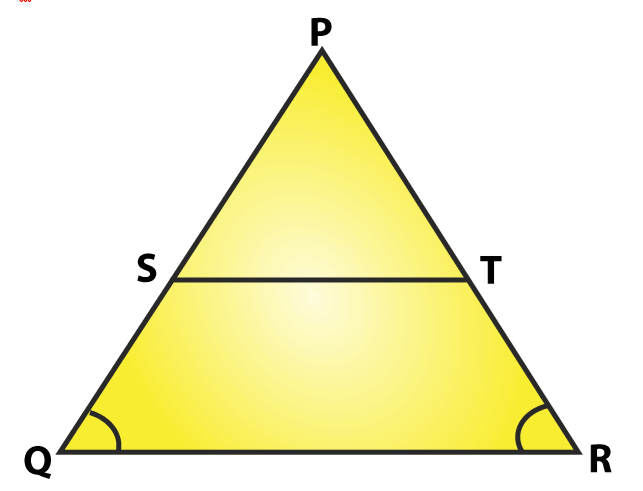Since, $\mathrm{PQ}=\mathrm{PR}$, so $\triangle \mathrm{PQR}$ is an isosceles triangle.

$\angle P Q R=\angle P R Q$

Now, $\angle P S T=\angle P Q R$ and $\angle P T S=\angle P R Q$

[Corresponding angles as ST parallel to $\mathrm{QR}]$

Since, $\angle \mathrm{PQR}=\angle \mathrm{PRQ}$

$\angle \mathrm{PST}=\angle \mathrm{PTS}$

In $\triangle$ PST,

$\angle \mathrm{PST}=\angle \mathrm{PTS}$

$\triangle \mathrm{PST}$ is an isosceles triangle.

Therefore, $\mathrm{PS}=\mathrm{PT}$

Hence proved.

Exercise $10.4$

Question 1: In figure, It is given that $A B=C D$ and $A D=B C$. Prove that $\triangle A D C \cong \Delta C B A$.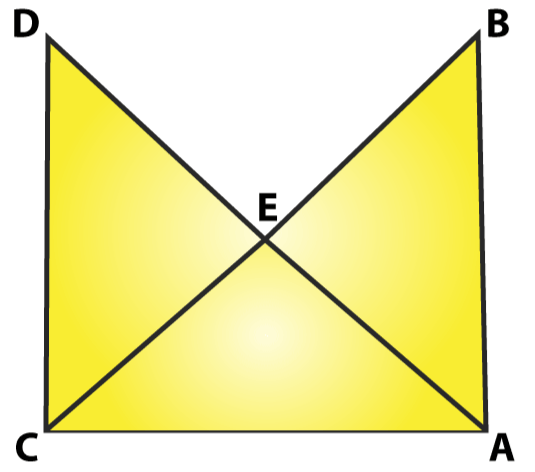Solution:

From figure, $A B=C D$ and $A D=B C$

To prove: $\triangle \mathrm{ADC} \cong \Delta \mathrm{CBA}$

Consider $\triangle \mathrm{ADC}$ and $\triangle \mathrm{CBA}$.

$\mathrm{AB}=\mathrm{CD}[\mathrm{Given}]$

$\mathrm{BC}=\mathrm{AD}[\mathrm{Given}]$

And $\mathrm{AC}=$ AC [Common side]

So, by SSS congruence criterion, we have

$\triangle \mathrm{ADC} \cong \Delta \mathrm{CBA}$

Hence proved.

Question 2: In a $\Delta \mathrm{PQR}$, if $\mathrm{PQ}=\mathrm{QR}$ and $\mathrm{L}, \mathrm{M}$ and $\mathrm{N}$ are the mid-points of the sides $\mathrm{PQ}, \mathrm{QR}$ and RP respectively. Prove that LN = MN.

Solution: Given: In $\triangle P Q R, P Q=Q R$ and $L, M$ and $N$ are the mid-points of the sides $P Q, Q R$ and $R P$ respectively

To prove: $L N=M N$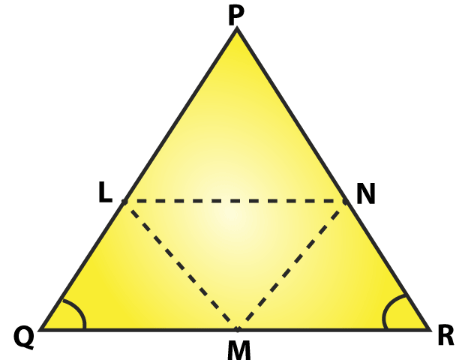Join $\mathrm{L}$ and $\mathrm{M}, \mathrm{M}$ and $\mathrm{N}, \mathrm{N}$ and $\mathrm{L}$

We have $\mathrm{PL}=\mathrm{LQ}, \mathrm{QM}=\mathrm{MR}$ and $\mathrm{RN}=\mathrm{NP}$

[Since, $L, M$ and $N$ are mid-points of $P Q, Q R$ and $R P$ respectively] And also $\mathrm{PQ}=\mathrm{QR}$

$P L=L Q=Q M=M R=P N=L R \ldots \ldots$(i)

[ Using mid-point theorem]

$\mathrm{MN} \| \mathrm{PQ}$ and $\mathrm{MN}=\mathrm{PQ} / 2$

$\mathrm{MN}=\mathrm{PL}=\mathrm{LQ} \ldots \ldots$(ii)

Similarly, we have

$\mathrm{LN} \| \mathrm{QR}$ and $\mathrm{LN}=(1 / 2) \mathrm{QR}$

$\mathrm{LN}=\mathrm{QM}=\mathrm{MR} \ldots \ldots$(iii)

From equation (i), (ii) and (iii), we have

$\mathrm{PL}=\mathrm{LQ}=\mathrm{QM}=\mathrm{MR}=\mathrm{MN}=\mathrm{LN}$

This implies, $L N=M N$

Hence Proved.

Exercise $10.5$

Question 1: $A B C$ is a triangle and $D$ is the mid-point of $B C$. The perpendiculars from $D$ to $A B$ and $\mathrm{AC}$ are equal. Prove that the triangle is isosceles.

Solution: Given: $\mathrm{D}$ is the midpoint of $\mathrm{BC}$ and $\mathrm{PD}=\mathrm{DQ}$ in a triangle $\mathrm{ABC}$.

To prove: $A B C$ is isosceles triangle.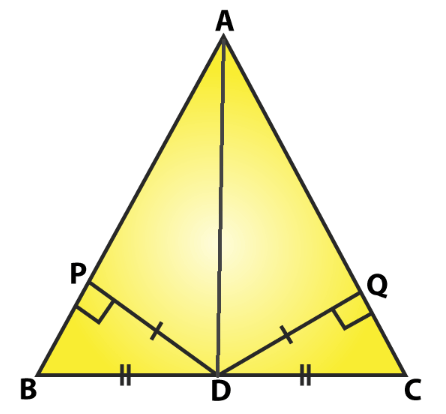In $\triangle B D P$ and $\triangle C D Q$

$\mathrm{PD}=\mathrm{QD}$ (Given)

$B D=D C(D$ is mid-point $)$

$\angle B P D=\angle C Q D=90^{\circ}$

By RHS Criterion: $\triangle \mathrm{BDP} \cong \triangle \mathrm{CDQ}$

$\mathrm{BP}=\mathrm{CQ} \ldots$ (i) (By $\mathrm{CPCT})$

In $\triangle \mathrm{APD}$ and $\triangle \mathrm{AQD}$

$\mathrm{PD}=\mathrm{QD}$ (given)

$\mathrm{AD}=\mathrm{AD}(\mathrm{common})$

$\mathrm{APD}=\mathrm{AQD}=90^{\circ}$

By RHS Criterion: $\triangle \mathrm{APD} \cong \triangle \mathrm{AQD}$

So, $P A=Q A \ldots$ (ii) $($ By $C P C T)$

$\mathrm{BP}+\mathrm{PA}=\mathrm{CQ}+\mathrm{QA}$

$\mathrm{AB}=\mathrm{AC}$

Two sides of the triangle are equal, so $\mathrm{ABC}$ is an isosceles.

Question 2: $A B C$ is a triangle in which BE and CF are, respectively, the perpendiculars to the sides $A C$ and $A B$. If $B E=C F$, prove that $\Delta A B C$ is isosceles

Solution:

$\mathrm{ABC}$ is a triangle in which $\mathrm{BE}$ and $\mathrm{CF}$ are perpendicular to the sides $\mathrm{AC}$ and $\mathrm{AB}$ respectively s.t. BE $=\mathrm{CF}$.

To prove: $\triangle \mathrm{ABC}$ is isosceles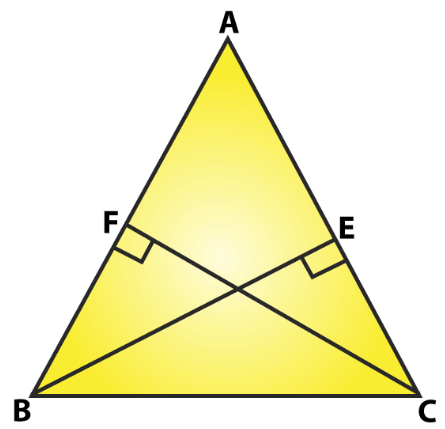In $\triangle \mathrm{BCF}$ and $\triangle \mathrm{CBE}$,

$\angle B F C=C E B=90^{\circ}$ [Given]

$\mathrm{BC}=\mathrm{CB}$ [Common side]

And $\mathrm{CF}=\mathrm{BE}$ [Given]

By RHS congruence criterion: $\triangle \mathrm{BFC} \cong \Delta \mathrm{CEB}$

So, $\angle \mathrm{FBC}=\angle \mathrm{EBC}[\mathrm{By} \mathrm{CPCT}]$

$=>\angle \mathrm{ABC}=\angle \mathrm{ACB}$

$A C=A B$ [Opposite sides to equal angles are equal in a triangle]

Two sides of triangle $A B C$ are equal.

Therefore, $\triangle \mathrm{ABC}$ is isosceles. Hence Proved.

Question 3: If perpendiculars from any point within an angle on its arms are congruent. Prove that it lies on the bisector of that angle.

Solution: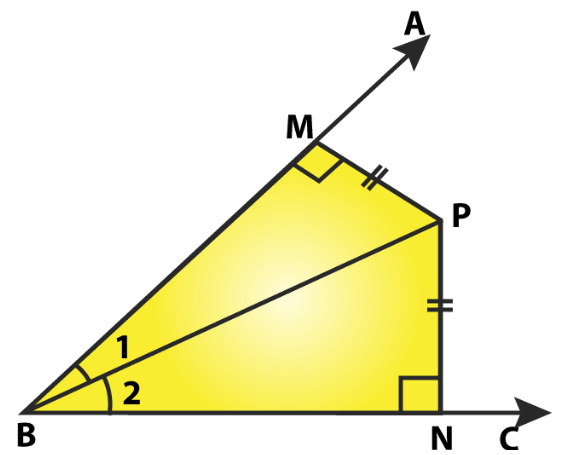Consider an angle $\mathrm{ABC}$ and $\mathrm{BP}$ be one of the arm within the angle.

Draw perpendiculars PN and PM on the arms BC and BA.

In $\triangle \mathrm{BPM}$ and $\triangle \mathrm{BPN}$,

$\angle \mathrm{BMP}=\angle \mathrm{BNP}=90^{\circ}$ [given]

$\mathrm{BP}=\mathrm{BP} \text { [Common side] }$

MP = NP [given]

By RHS congruence criterion: $\triangle \mathrm{BPM} \cong \triangle \mathrm{BPN}$

So, $\angle M B P=\angle N B P$ [ By CPCT]

BP is the angular bisector of $\angle A B C$.

Hence proved

Exercise $10.6$ Page No: $10.66$

Question 1: In $\triangle \mathrm{ABC}$, if $\angle \mathrm{A}=40^{\circ}$ and $\angle \mathrm{B}=60^{\circ} .$ Determine the longest and shortest sides of the triangle.

Solution: In $\triangle \mathrm{ABC}, \angle \mathrm{A}=40^{\circ}$ and $\angle \mathrm{B}=60^{\circ}$

We know, sum of angles in a triangle $=180^{\circ}$

$\angle A+\angle B+\angle C=180^{\circ}$

$40^{\circ}+60^{\circ}+\angle C=180^{\circ}$

$\angle C=180^{\circ}-100^{\circ}=80^{\circ}$

$\angle C=80^{\circ}$

Now, $40^{\circ}<60^{\circ}<80^{\circ}$

$=>A<\angle B<\angle C$

$=>\angle C$ is greater angle and $\angle A$ is smaller angle.

Now, $\angle A<\angle B<\angle C$

$\Rightarrow \angle A<\angle B<\angle C$

$=>\angle C$ is greater angle and $\angle A$ is smaller angle.

Now, $\angle A<\angle B<\angle C$

We know, side opposite to greater angle is larger and side opposite to smaller angle is smaller.

Therefore, AB is greater than AC, AC is greater than BC

$\mathrm{AB}$ is longest and $\mathrm{BC}$ is shortest side.

Question 2: In a $\triangle \mathrm{ABC}$, if $\angle \mathrm{B}=\angle \mathrm{C}=45^{\circ}$, which is the longest side?

Solution: $\ln \triangle \mathrm{ABC}, \angle \mathrm{B}=\angle \mathrm{C}=45^{\circ}$

Sum of angles in a triangle $=180^{\circ}$

$\angle A+\angle B+\angle C=180^{\circ}$

$\angle A+45^{\circ}+45^{\circ}=180^{\circ}$

$\angle A=180^{\circ}-\left(45^{\circ}+45^{\circ}\right)$

$=180^{\circ}-90^{\circ}=90^{\circ}$

$\angle A=90^{\circ}$

$\Rightarrow \angle B=\angle C<\angle A$

Therefore, $B C$ is the longest side.

Question 3: In $\triangle \mathrm{ABC}$, side $\mathrm{AB}$ is produced to $\mathrm{D}$ so that $\mathrm{BD}=\mathrm{BC}$. If $\angle \mathrm{B}=60^{\circ}$ and $\angle \mathrm{A}=70^{\circ}$.

Prove that: (i) $A D>C D$ (ii) $A D>A C$

Solution: $\ln \triangle \mathrm{ABC}$, side $\mathrm{AB}$ is produced to $\mathrm{D}$ so that $\mathrm{BD}=\mathrm{BC}$.

$\angle B=60^{\circ}$, and $\angle A=70^{\circ}$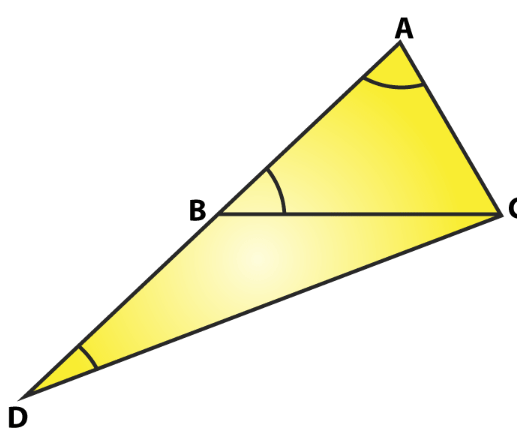To prove: (i) $A D>C D$ (ii) $A D>A C$

Construction: Join C and D

We know, sum of angles in a triangle $=180^{\circ}$

$\angle A+\angle B+\angle C=180^{\circ}$

$70^{\circ}+60^{\circ}+\angle C=180^{\circ}$

$\angle C=180^{\circ}-\left(130^{\circ}\right)=50^{\circ}$

$\angle C=50^{\circ}$

$\angle A C B=50^{\circ} \ldots \ldots($ i $)$

And also in $\triangle B D C$

$\angle \mathrm{DBC}=180^{\circ}-\angle \mathrm{ABC}=180-60^{\circ}=120^{\circ}$

[\angleDBA is a straight line]

and $B D=B C$ [given]

$\angle B C D=\angle B D C$ [Angles opposite to equal sides are equal]

Sum of angles in a triangle $=180^{\circ}$

$\angle \mathrm{DBC}+\angle \mathrm{BCD}+\angle \mathrm{BDC}=180^{\circ}$

$120^{\circ}+\angle B C D+\angle B C D=180^{\circ}$

$120^{\circ}+2 \angle \mathrm{BCD}=180^{\circ}$

$2 \angle \mathrm{BCD}=180^{\circ}-120^{\circ}=60^{\circ}$

$\angle B C D=30^{\circ}$

$\angle B C D=\angle B D C=30^{\circ} \ldots$(ii)

Now, consider $\triangle$ ADC.

$\angle \mathrm{DAC}=70^{\circ}$ [given]

$\angle A D C=30^{\circ}$ [From (ii)]

$\angle A C D=\angle A C B+\angle B C D=50^{\circ}+30^{\circ}$

$=80^{\circ}$ [From (i) and (ii)]

Now, $\angle A D C<\angle D A C<\angle A C D$

$A C [Side opposite to greater angle is longer and smaller angle is smaller]$A D>C D$and$A D>A C$Hence proved. Question 4: Is it possible to draw a triangle with sides of length$2 \mathrm{~cm}, 3 \mathrm{~cm}$and$7 \mathrm{~cm}$? Solution: Lengths of sides are$2 \mathrm{~cm}, 3 \mathrm{~cm}$and$7 \mathrm{~cm}$. A triangle can be drawn only when the sum of any two sides is greater than the third side. So, let's check the rule.$2+3 \not>7$or$2+3<72+7>3$and$3+7>2$Here$2+3 \not>7$So, the triangle does not exit. Exercise VSAQs Question 1 : In two congruent triangles$\mathrm{ABC}$and$\mathrm{DEF}$, if$\mathrm{AB}=\mathrm{DE}$and$\mathrm{BC}=\mathrm{EF}$. Name the pairs of equal angles. Solution: In two congruent triangles$A B C$and DEF, if$A B=D E$and$B C=E F$, then$\angle A=\angle D, \angle B=\angle E$and$\angle C=\angle F$Question 2: In two triangles$A B C$and DEF, it is given that$\angle A=\angle D, \angle B=\angle E$and$\angle C=\angle F$. Are the two triangles necessarily congruent? Solution: No. Reason: Two triangles are not necessarily congruent, because we know only angle-angle-angle (AAA) criterion. This criterion can produce similar but not congruent triangles. Question 3: If$A B C$and DEF are two triangles such that$A C=2.5 \mathrm{~cm}, B C=5 \mathrm{~cm}, C=75^{\circ}$, DE =$2.5 \mathrm{~cm}, \mathrm{DF}=5 \mathrm{~cm}$and$\mathrm{D}=75^{\circ}$. Are two triangles congruent? Solution: Yes. Reason: Given triangles are congruent as$\mathrm{AC}=\mathrm{DE}=2.5 \mathrm{~cm}, \mathrm{BC}=\mathrm{DF}=5 \mathrm{~cm}$and$\angle D=\angle C=75^{\circ}$. By SAS theorem triangle$\mathrm{ABC}$is congruent to triangle EDF. Question 4: In two triangles$A B C$and$A D C$, if$A B=A D$and$B C=C D$. Are they congruent? Solution: Yes. Reason: Given triangles are congruent as$\mathrm{AB}=\mathrm{AD}\mathrm{BC}=\mathrm{CD}$and AC [ common side] By SSS theorem triangle$A B C$is congruent to triangle$A D C$. Question 5: In triangles$A B C$and$C D E$, if$A C=C E, B C=C D, \angle A=60^{\circ}, \angle C=30^{\circ}$and$\angle D=90^{\circ}$Are two triangles congruent? Solution: Yes. Reason: Given triangles are congruent Here$A C=C EB C=C D\angle B=\angle D=90^{\circ}$By SSA criteria triangle$A B C$is congruent to triangle$C D E .$Question 6:$\mathrm{ABC}$is an isosceles triangle in which$\mathrm{AB}=$AC. BE and CF are its two medians. Show that$B E=C F$Solution:$A B C$is an isosceles triangle (given)$\mathrm{AB}=\mathrm{AC}$(given) BE and CF are two medians (given) To prove:$\mathrm{BE}=\mathrm{CF}$In$\triangle \mathrm{CFB}$and$\triangle \mathrm{BEC}\mathrm{CE}=\mathrm{BF}($Since,$\mathrm{AC}=\mathrm{AB}=A C / 2=A B / 2=C E=B F)\mathrm{BC}=\mathrm{BC}$(Common)$\angle \mathrm{ECB}=\angle \mathrm{FBC}$(Angle opposite to equal sides are equal) By SAS theorem:$\triangle \mathrm{CFB} \cong \triangle \mathrm{BEC}$So,$B E=C F\$ (By c.p.c.t)